### Geometry I

The figure below is a regular hexagon dissected into a triangle, a rhombus, and a trapezoid.

If the area of the blue triangle is $3,$ what is the area of the red trapezoid?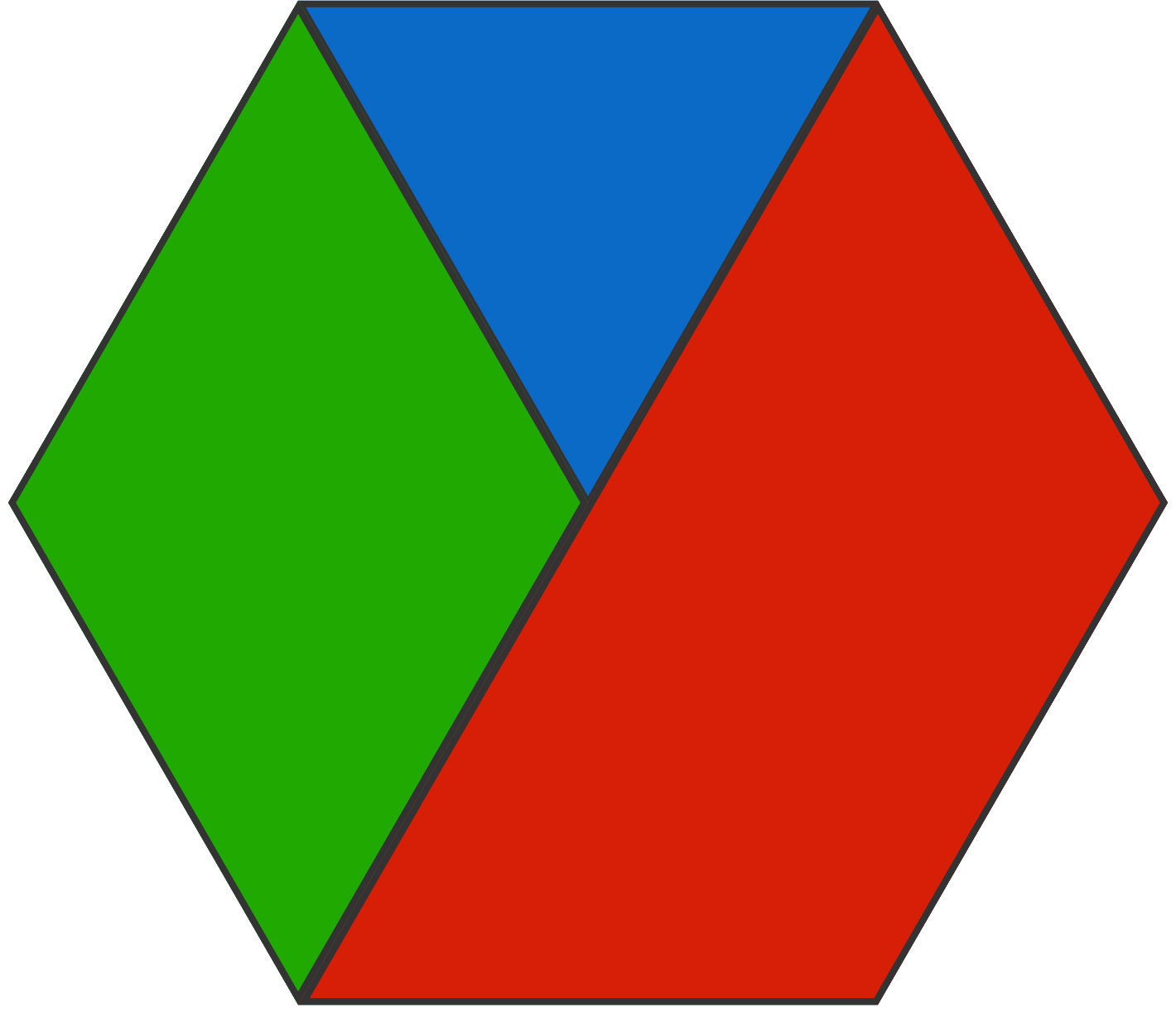# Triangles and Hexagons

Which has a greater area: an equilateral matchstick triangle made out of 12 matchsticks, or a regular hexagon with the same perimeter?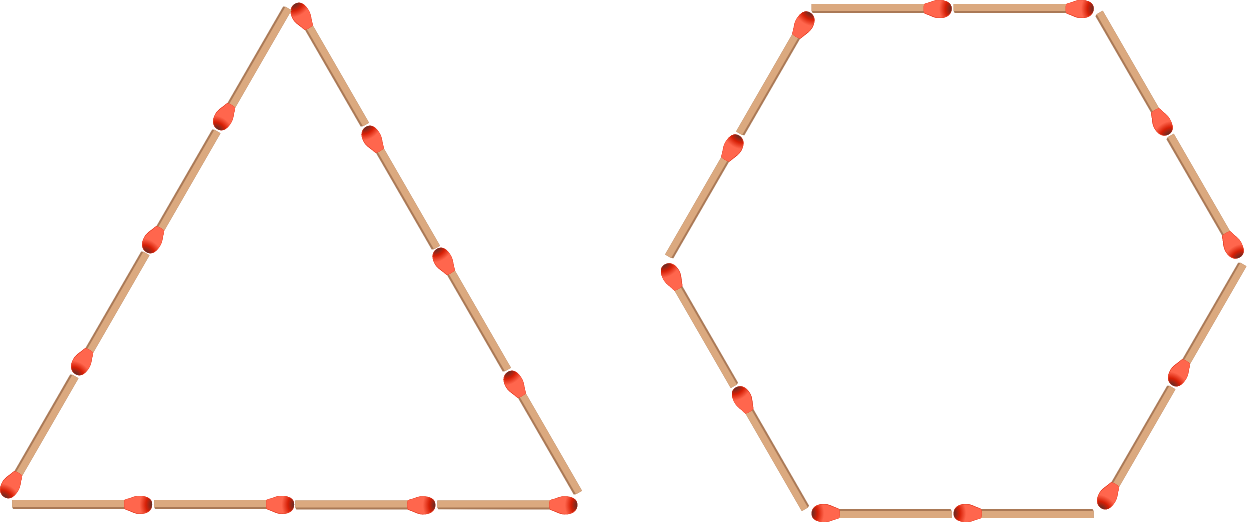# Triangles and Hexagons

Try this riddle:

I am a peculiar quadrilateral. If you put 3 copies of me together, I can form an equilateral triangle. Similarly, if you put 3 copies of an equilateral triangle together, then they can form me!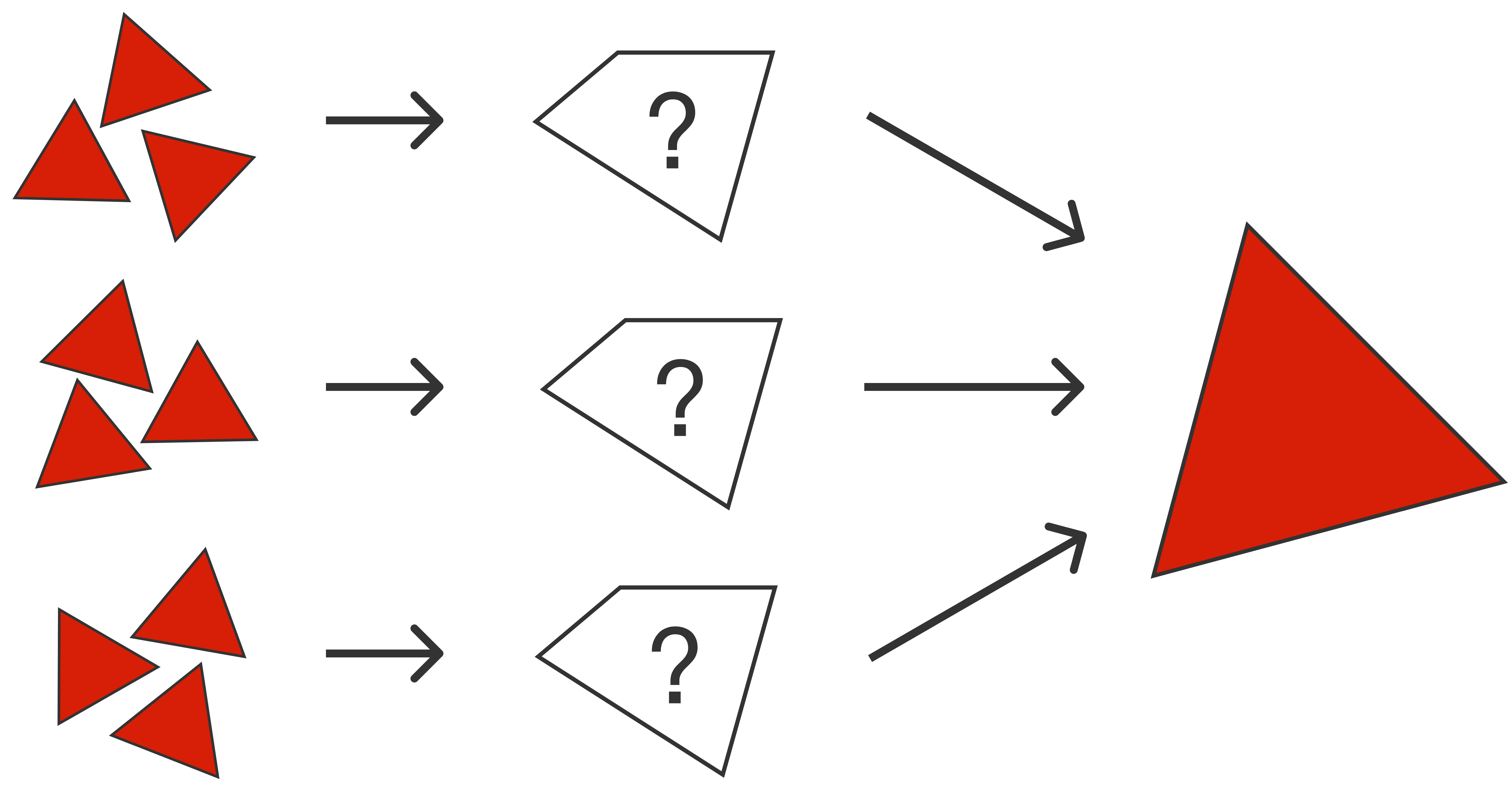Definitions:

• parallelogram
• trapezoid
• rhombus

# Triangles and Hexagons

An equilateral triangle is inscribed in a regular hexagon so that its vertices lie on three vertices of the hexagon, as shown below. Which is greater, the area of the red section or the area of the blue?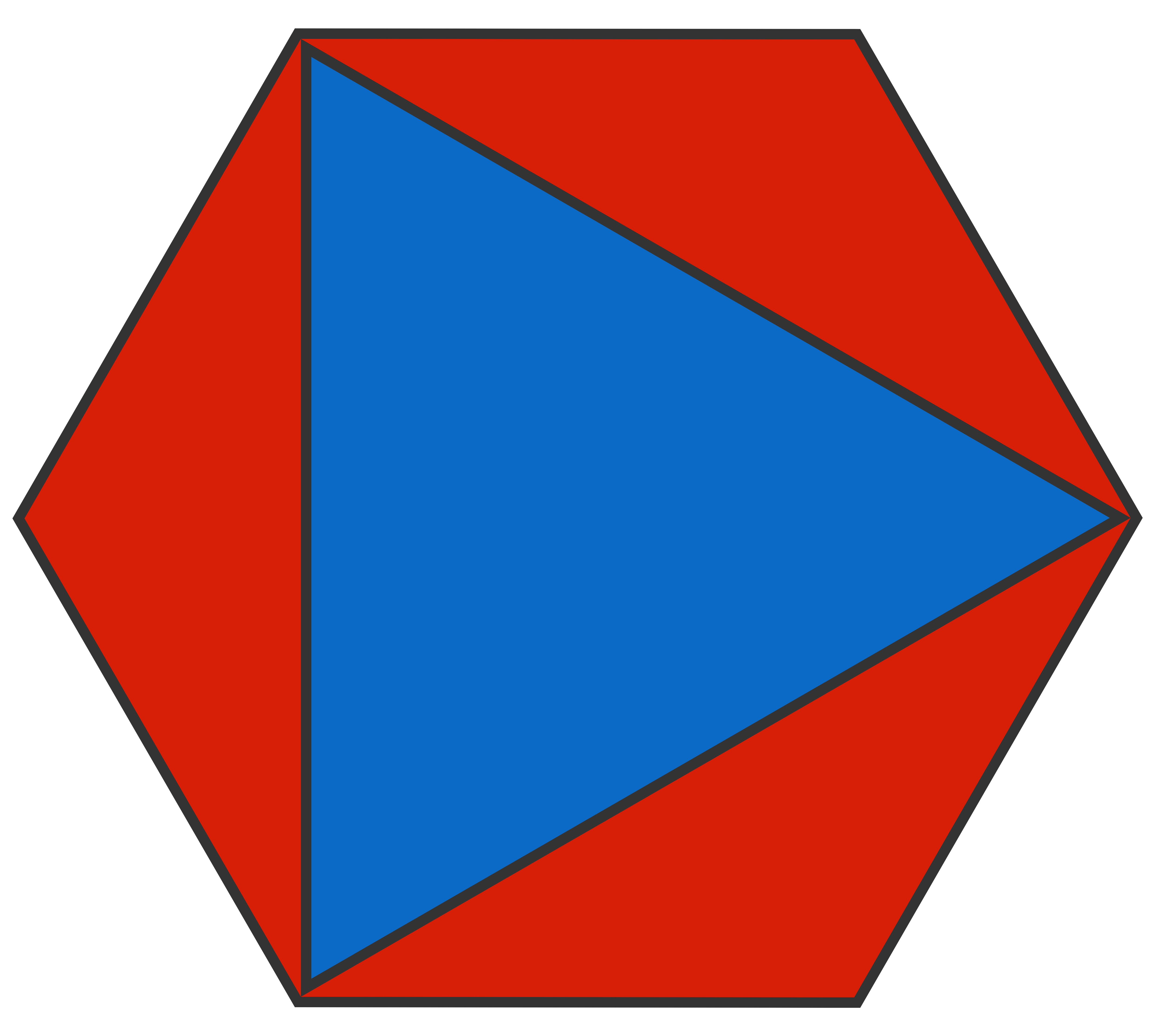# Triangles and Hexagons

An equilateral triangle is inscribed in a regular hexagon so that its vertices lie on the midpoints of 3 of the hexagon's sides, as shown below. Which is greater, the area of the red section or the area of the blue?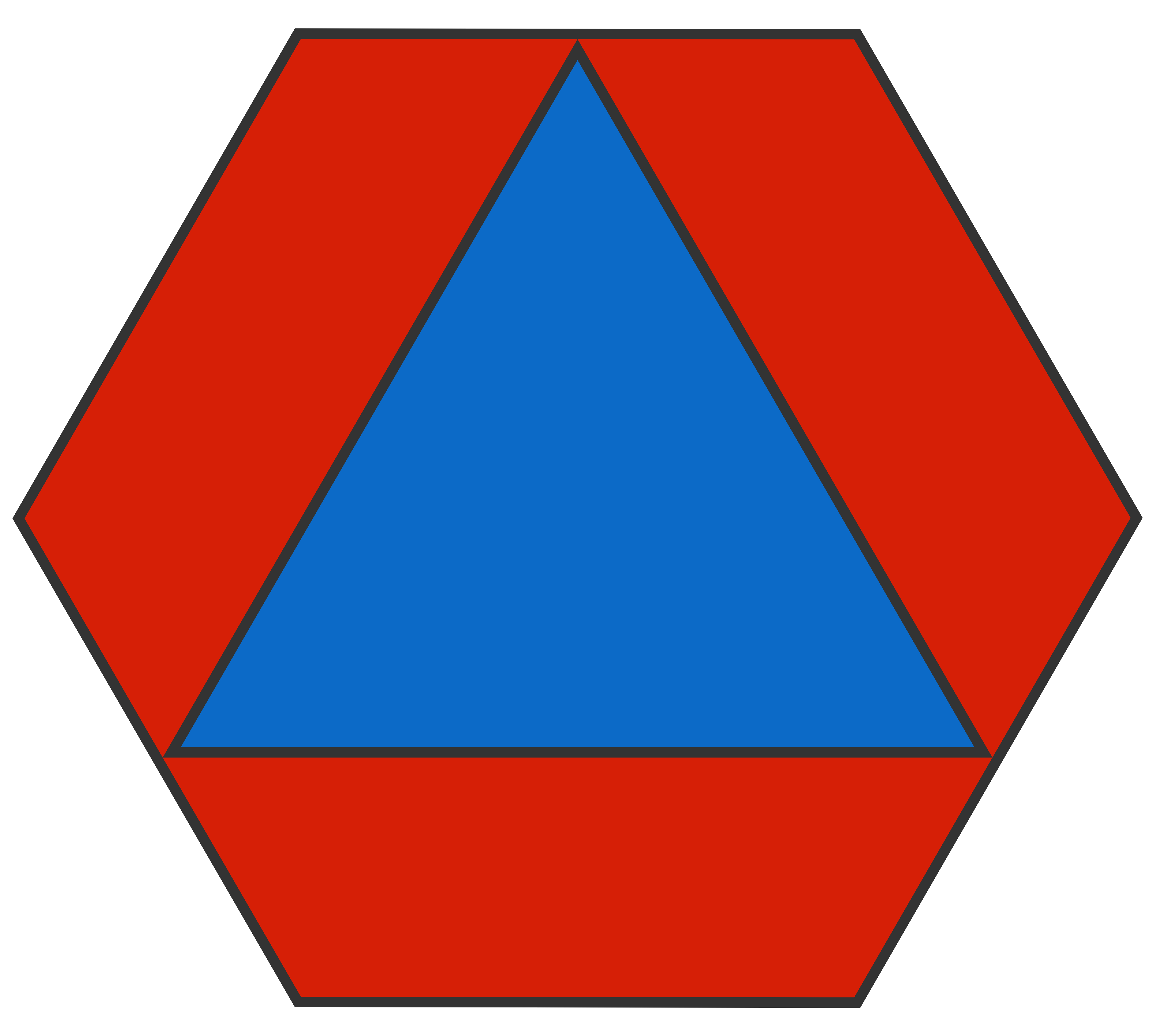×